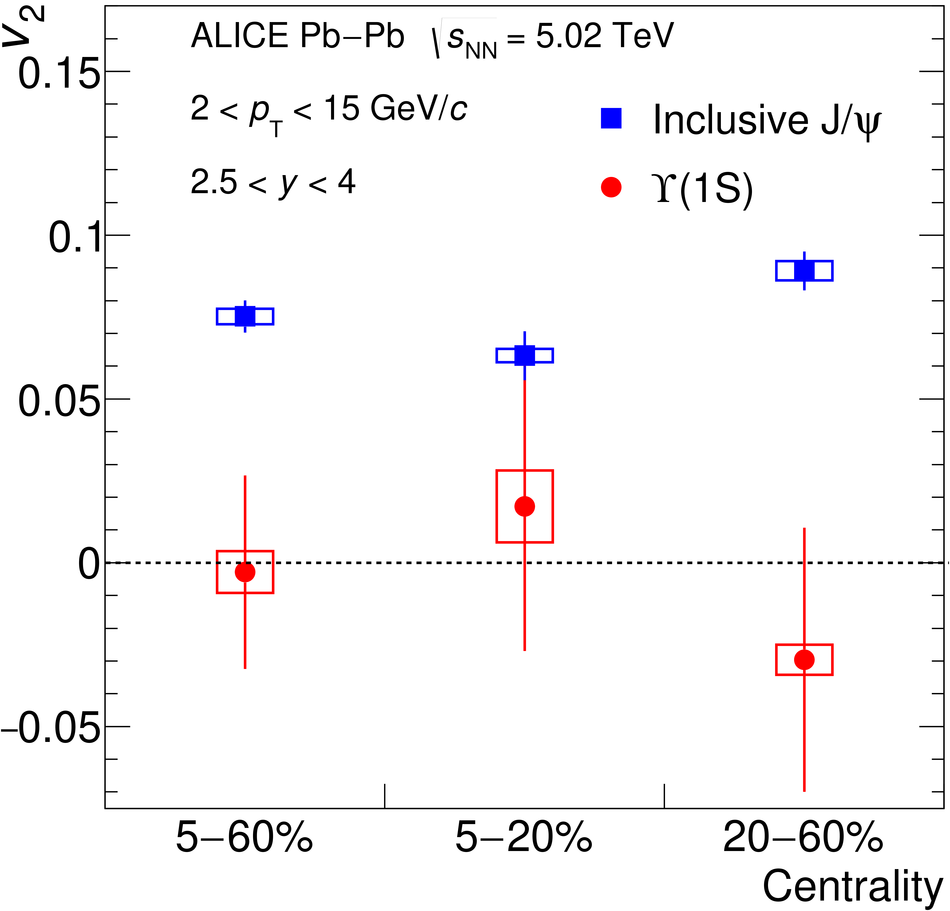# Measurement of $Υ(1{\rm S})$ elliptic flow at forward rapidity in Pb-Pb collisions at $\sqrt{s_{\rm{NN}}}=5.02$ TeV

The first measurement of the $\Upsilon(1{\rm S})$ elliptic flow coefficient ($v_2$) is performed at forward rapidity (2.5 $<~$ $y$ $<~$ 4) in Pb-Pb collisions at $\sqrt{s_{\rm NN}} = 5.02$ TeV with the ALICE detector at the LHC. The results are obtained with the scalar product method and are reported as a function of transverse momentum ($p_{\rm{T}}$) up to 15 GeV/$c$ in the 5-60% centrality interval. The measured $\Upsilon(1{\rm S})$ $v_2$ is consistent with zero and with the small positive values predicted by transport models within uncertainties. The $v_2$ coefficient in 2 $<~$ $p_{\rm T}$ $<~$ 15 GeV/$c$ is lower than that of inclusive J/$\psi$ mesons in the same $p_{\rm{T}}$ interval by 2.6 standard deviations. These results, combined with earlier suppression measurements, are in agreement with a scenario in which the $\Upsilon$(1S) production in Pb-Pb collisions at LHC energies is dominated by dissociation limited to the early stage of the collision whereas in the J/$\psi$ case there is substantial experimental evidence of an additional regeneration component.

Figures

## Figure 1

 Left: The $M_{\mu\mu}$ distribution in the 5--60\ extended Crystal Ball function for the signal and a Variable-Width Gaussian function for the background. Right: The $v_2(M_{\mu\mu})$ distribution in the same centrality and $p_{\rm T}$ intervals fitted with the function from Eq.~(\ref{eq:v2_sb}).## Figure 2## Figure 3

 The $\Upsilon$(1S) $v_2$ coefficient integrated over the transverse momentum range 2 $< \pt <$ 15 GeV/$c$ in three centrality intervals compared to that of inclusive ${\rm J}/\psi$. Error bars (open boxes) represent the statistical (systematic) uncertainties.